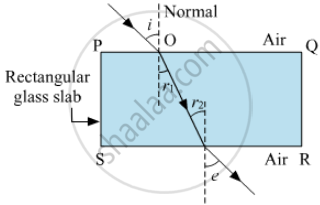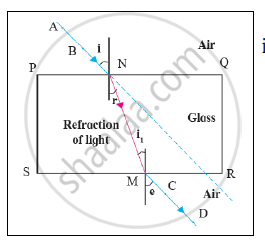# Prove the Statement . If the Angle of Incidence and Angle of Emergence of a Light Ray Falling on a Glass Slab Are I and E Respectively, Prove That, I = E. - Science and Technology 1

Diagram

Prove the statement .

If the angle of incidence and angle of emergence of a light ray falling on a glass slab are i and e respectively, prove that, i = e.

#### Solution 1Let $\mu$ be the refractive index of the glass slab.  Then, according to Snell's law,

$\frac{\sin i}{\sin r_1} = \mu$      .....(i)

and
$\frac{\sin r_2}{\sin e} = \frac{1}{\mu}$   .....(ii)
But, r1 = r2   .....(iii)
Putting (iii) in (i), we have
$\frac{\sin r_2}{\sin e} = \mu$      .....(iv)
Multiplying (i) and (iv), we have
$\frac{\sin i}{\sin e} = 1$
or
$i = e$

#### Solution 2in figure. PQ || SR
NM is a refracted ray. ∴ 𝑟= 𝑖1
By the laws of refraction,
𝑔𝑛𝑎= (sin i)/(sin r) ;  _a n_g= (sin i _1)/(sin_e)
 therefore  _g n_a  = 1 / (" _a n_g)
∴ (sin i)/(sin r)  = (sin e)/(sin i _1)    .......but  r=i_1
∴  sin 𝑖 = sin 𝑒
∴  𝑖 = 𝑒

Concept: Refractive Index
Is there an error in this question or solution?